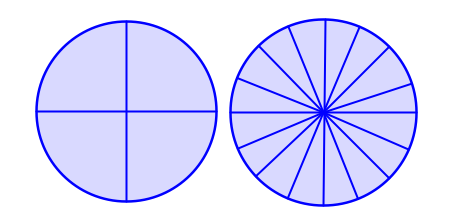### Home > MC1 > Chapter 7 > Lesson 7.2.2 > Problem7-55

7-55.

Which is greater, $\frac { 3 } { 4 }$ or $\frac { 11 } { 16 }$? How can you be sure?

$\text{If you can find how many }\frac{1}{16}\text{'s are equal to }\frac{3}{4}\text{ you might be able to find which is greater.}$

Also, Julia drew this picture to solve this problem.$\text{Can you draw your own picture and fill in }\frac{3}{4}\text{ and }\frac{11}{16}?$
Now compare the two portions and see which is larger.

Which fraction is greater? Make sure to show or describe how you know!